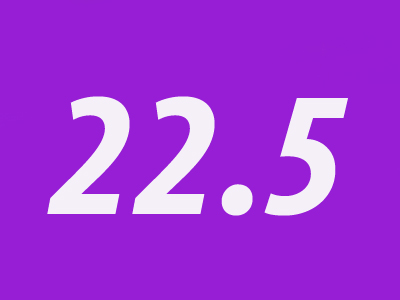25% of 90 is 22.5

# Percentages 3 (Medium)

Percentages are a way of representing parts of numbers, similar to fractions or decimals. For your Eleven Plus maths exam you will have to calculate percentages. If you haven’t mastered it yet then don’t worry – this quiz will show you how to do it.

Let’s give you an example:

What is 25% of 90? Well, first of all, divide 90 by 100 to work out 1% of 90:
90 ÷ 100 = 0.9
We want to find 25% of 90 so next we multiply 0.9 by 25:
0.9 x 25 = 22.5 so, 25% of 90 is 22.5.

I hope that’s made things easier to understand. Have a go at this quiz and (if you haven’t already) play our other quizzes on percentages. The more you practise, the easier they will be.

1.
What is 27% of 95?
25.65
26.55
26.65
27
27% of 95 = (27 ÷ 100) × 95 = (27 × 95) ÷ 100 = 25.65.
DON'T forget: when you divide by 100, move the digits two places to the right
2.
What is 5% of 5% of 200?
5
0.5
10
2
5% of 200 = 10
5% of 10 = 0.5
3.
What is 32.5% of 120?
35
37
39
41
32.5% of 120 = (32.5 ÷ 100) × 120 = (32.5 × 120) ÷ 100 = 39
DON'T forget: when you divide by 100, move the digits two places to the right
4.
What is 7.5% of 90?
5.75
6
6.75
7
7.5% of 90 = (7.5 ÷ 100) × 90 = (7.5 × 90) ÷ 100 = 6.75
5.
Stuart has 64 marbles but loses 25% of them. How many marbles does he have now?
16
48
18
50
25% of 64 = (25 ÷ 100) × 64 = (25 × 64) ÷ 100 = 16
Now we must subtract 16 from 64 to find the answer: 64 - 16 = 48
6.
Brandon’s business makes a 20% profit. He spent £5,000 on his business, so how much were his profits worth?
£6,000
£2,500
£500
£1,000
To find this answer we need to know what 20% of £5,000 is:
5,000 ÷ 100 = 50
50 x 20 = 1,000. Brandon made £1,000 in profits
7.
Michael’s house was worth £205,000 when he bought it last year. Since then its value has increased by 3%. How much is Michael’s house worth now?
£208,000
£209,500
£210,250
£211,150
3% of £205,000 = (3 ÷ 100) × 205,000 = (3 × 205,000) ÷ 100 = £6,150
This is the amount that Michael’s house value has increased by. So, Michael’s house is now worth £205,000 + £6,150
£205,000 + £6,150 = £211,150
8.
What is 40% of 19?
9.2
8.4
7.6
6.8
19 ÷ 100 = 0.19
0.19 x 40 = 7.6
9.
In a general election, 31 million people voted. If 40% voted for the Blue Party, how many people did not vote for the Blue Party?
12.4 million
60%
18.6 million
40%
40% of 31 million = (40 ÷ 100) × 31 million = (40 × 31 million) ÷ 100 = 12.4 million.
That’s how many people voted for the Blue Party. To work out how many did not vote for the Blue party, you need to subtract 12.4 million from 31 million:
31 - 12.4 = 18.6 so, 18.6 million people did not vote for the Blue Party - that's 60% of the voters, but you were asked for the number of people, not the percentage. Beware of questions like this!
10.
What is 90% of 1,000?
900
990
910
999
90% of 100 is 90, so 90% of 1,000 will be 900. Just times by 10
Author:  Frank Evans